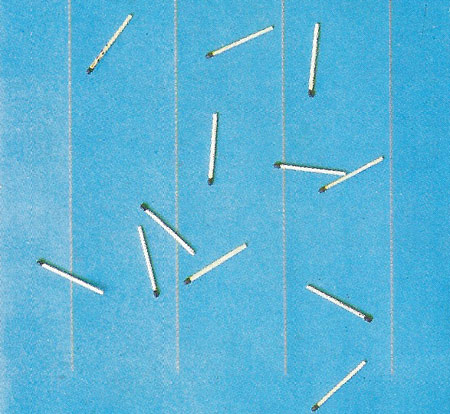A# Buffon's needleIn the Buffon needle problem, a match is thrown at random on a striped cloth. If the stripes are n match-lengths wide the chance of it coming to rest across a line is 2/(nπ). It is surprising to find π in this answer: it enters because the match can lie at any angle; like a spoke in a wheel thrown onto the stripes.

Buffon's needle is an early problem in geometrical probability that was investigated experimentally in 1777 by the French naturalist and mathematician Comte Georges Louis de Buffon. It involves dropping a needle repeatedly onto a lined sheet of paper and finding the probability of the needle crossing one of the lines on the page. The result, surprisingly, is directly related to the value of pi.

Consider a simple case in which the lines are 1 cm apart and the needle is 1 cm in length. After many drops the probability of the needle lying across a line is found to be very close to 2/π. Why? There are two variables: the distance from the center of the needle to the closest line, d, which can vary between 0 and 0.5 cm, and the angle, θ, at which the needle falls with respect to the lines, which can be vary between 0 to 180°. The needle will hit a line if d ≤ ½ sin θ. In a plot of d against ½ sin θ, the values on or below the curve represent a hit; thus, the probability of a success is the ratio of the area below the curve to the area of entire rectangle. The area below the curve is given by the integral of ½ sin θ from 0 to π, which is π. The area of the rectangle is π. So, the probability of a hit is 1/(π/2) or 2/π (about 0.637). Dropping a needle many times on to lined paper gives an interesting (but slow) way to find π.

This kind of probabilistic means of performing calculations is the basis of a technique known as the Monte Carlo method.